# Automatic Chart Harmonisation

By

Ray BlakeWhy Excel is missing a charting feature and how that feature can be recreated using VBA.

Excel's charting tools are pretty comprehensive, but there are some annoying features missing. This article will look at one of them – the automatic harmonisation of scales – and build some code to work around the issue. I will assume a good working knowledge of how charts are created and customised in Excel.

Throughout the article, we'll be using the following data table, relating to survey data in a chain store:

Fairly unhappy customers Very unhappy customers
Aberdeen 50 55
Edinburgh 50 120
Glasgow 300 140

When you autochart these numbers as a separate chart for each store, here is what you get: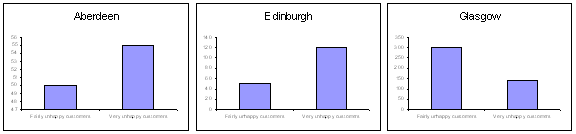If every picture tells a story, this is an odd one. Notice how Aberdeen's and Edinburgh's figures appear the same, whilst the latter has more than twice as many Very Unhappy Customers (VUCs). Glasgow looks to be performing much better than either of the others, too, but it has the most VUCs of any of them, and some six times as many Fairly Unhappy Customers, too!

Excel has applied automatic scales. It has looked at the data you wanted to chart, and has made assumptions about where you want the Y scale to start and end. For instance, it has to chart for Aberdeen values of 50 and 55. It sets up a scale ranging from 47 to 56. When looking to chart values of 300 and 140 for Glasgow, though, it uses a scale of 0 to 350. If you don't look carefully at the scales, you might draw an unwise conclusion when comparing these charts.

Right now, many of you will be wondering why I didn't just show a single graph and plot all 3 sets of figures on the same one, like this: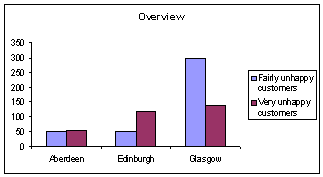Actually, there are a number of possible reasons. One might be that I want to copy and paste individual charts into letters to the store managers, but I might want each only to have access to the figures for his/her own store.

One way I can solve the problem of different scales on the 3 graphs is to manually set the scales on each chart. Double-clicking on the Y axis line brings up the following dialog box: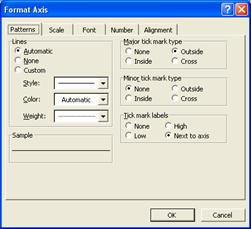If I hit the 'Scale' tab, I have access to these controls: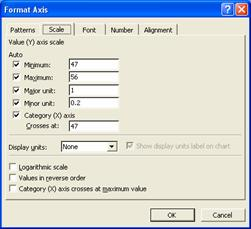I can remove the ticks from 'Minimum' and 'Maximum' and show their values as 0 and 320 respectively. If I do this for each of the 3 charts, here's what I get: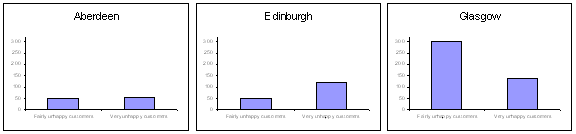That's more like it. You can make a reliable assessment now at a glance. But this comes at a price. By removing the automatic scaling, I have lost the ability to have the charts automatically cope with varying data. For instance, assume that some more data is added so that the figures are increased:

Fairly unhappy customers Very unhappy customers
Aberdeen 160 355
Edinburgh 70 180
Glasgow 500 200

Look what happens to the charts: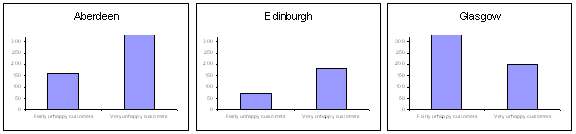Notice that the top of the scale is stuck at 320, so figures greater than this just get cut off. Not good. If we just reinstate the automatic scaling, we're back to Square 1, with each chart choosing its own scale independently. What we need is a way to harmonise automatic scaling between the 3 charts, and it's here that Excel lets us down.

What we can do, though, is fake it by using some VBA code. First, I need to add a couple of fields to the Excel sheet, thus: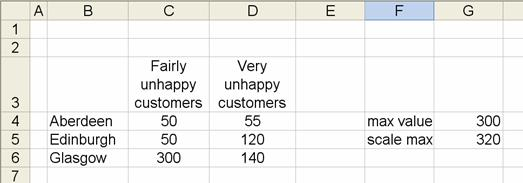The formula in cell G4 reads:

``=MAX(C4:D6)``

This finds the biggest value which exists in the data table.

IN G5, the formula is:

``=CEILING(G4+1,20)``

What this formula does is start with the number calculated in the cell above. It then:

2. Rounds up to the nearest multiple of 20

The end result is that it returns a round number up to 20 bigger than the largest number in the data.

If we assume the 3 charts on the worksheet are called "Chart 1", "Chart 2" and "Chart 3", then the VBA you need is as follows : (go to tools/macro/Visual Basic editor, then from Microsoft Visual Basic go to view/code)

``````Sub UpdateScale()
'
' by Ray Blake
'
ActiveSheet.ChartObjects("Chart 1").Activate
With ActiveChart.Axes(xlValue)
.MinimumScale = 0
.MaximumScale = Range("G5").Value
End With
ActiveSheet.ChartObjects("Chart 2").Activate
With ActiveChart.Axes(xlValue)
.MinimumScale = 0
.MaximumScale = Range("G5").Value
End With
ActiveSheet.ChartObjects("Chart 3").Activate
With ActiveChart.Axes(xlValue)
.MinimumScale = 0
.MaximumScale = Range("G5").Value
End With
End Sub``````

Whenever you run this code, the chart scales are updated to use the figure you calculated in Cell G5. You can run the code as a macro whenever more data is added, but a foolproof way of using it would be to insert a call to the code in the Worksheet Change event, thus:

``````Private Sub Worksheet_Change(ByVal Target As Range)